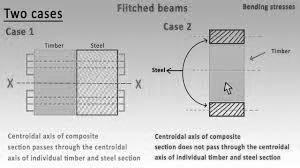Select Page

# Bending Stresses in Beams

Bending Stresses in Beams

Contents

Explain the concept of Bending 1

Define Simple Bending and Pure Bending 2

Explain the assumption made in the Theory of Simple Bending 3

Derive an expression for Bending Stresses 4

Explain the Neutral Axis 6

Define Moment of Resistance 6

Define Section Modulus 7

Describe and calculate Section Modulus for Rectangular and Hollow rectangular Section 8

Describe and calculate Section Modulus for Circular and Hollow circular Section 9

Describe and calculate Section Modulus for Square Section 9

Describe and calculate Section Modulus for Triangular Section 9

Describe and calculate Section Modulus for I (symmetrical and unsymmetrical) section 9

Describe and calculate Section Modulus for T-Section 9

Describe and calculate the Bending Stresses in Rectangular Section 9

Describe and calculate the Bending Stresses in Circular Section 9

Describe and calculate the Bending Stresses in Square Section 9

Describe and calculate Bending Stress in Triangular Section 9

Describe and calculate Bending Stresses in I (symmetrical and unsymmetrical) section 9

Describe and calculate Bending Stresses in T-section 9

Explain Comparison between Rectangular Beam 9

Explain Comparison between Square Beams 9

Explain Comparison between Rectangular and circular Beams 9

Explain the concept of Beam of Uniform Strength 9

Explain the phenomena of Eccentric Loading on the Cross-section of the Beam 9

Explain the Middle One-Third Rule for Rectangular Section 9

Explain the Middle One-Fourth Rule for Circular Section 9

Explain the concept of Composite Beams/Flitched Beam 9

# Explain the concept of Bending

Bending is a deformation that occurs when a force is applied to a structural element, such as a beam, causing it to change its shape. The force is typically perpendicular to the cross-section of the element, and results in a curvature along the length of the element. The amount of bending that occurs is determined by the magnitude of the applied force and the properties of the material, such as its elasticity and strength.

In engineering, the concept of bending is an important one, as it is a common mode of deformation in many structures, such as bridges, buildings, and other large-scale construction projects. Bending can result in significant stress and strain in the element, which can impact its strength, stability, and overall performance.

To understand the concept of bending, it is important to consider the internal forces and moments that occur within the element. When a force is applied to a beam, it creates both a shear force and a bending moment. The shear force acts to cut the beam along its length, while the bending moment acts to twist and bend the beam. The combination of these forces determines the amount of deformation that occurs, and the distribution of stress and strain within the element.

In practice, engineers use mathematical models and analytical techniques to predict and quantify the amount of bending that occurs in a structural element. This information is then used to design the element to withstand the loads and forces that it will be subject to, and to ensure that it will perform as intended. Overall, the concept of bending is a fundamental one in structural mechanics, and plays a critical role in the design and analysis of many engineering systems.

# Define Simple Bending and Pure Bending

Simple Bending:

Simple bending refers to the bending of a structural element, such as a beam, where the cross-section of the element remains plane and flat throughout the bending process. In simple bending, the normal stress (also known as the bending stress) is uniform along the length of the beam, and the shear stress is zero. Simple bending occurs when the loads applied to the beam are uniformly distributed along its length, and the axis of the beam remains straight.

Pure Bending:

Pure bending refers to a special case of simple bending where the applied load is a pure moment, or a twisting force. In pure bending, the cross-section of the beam remains plane and the axis of the beam remains straight, but the distribution of normal stress is not uniform. Instead, the normal stress is maximum at the top and bottom surfaces of the beam, and zero at the neutral axis. This type of bending is commonly encountered in engineering structures, such as bridges, where the loads are applied in a way that creates a twisting moment in the beam.

Both simple and pure bending are important concepts in structural mechanics, as they help engineers understand the behaviour and response of beams under different loading conditions. Understanding the difference between simple and pure bending is critical for correctly predicting and designing the behaviour of a structure, and for ensuring its safety and performance.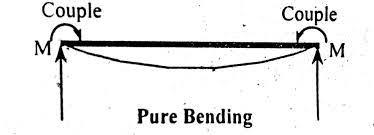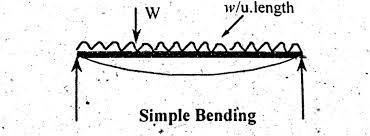# Explain the assumption made in the Theory of Simple Bending

The Theory of Simple Bending is a fundamental concept in structural mechanics that describes the behaviour of a beam under a load that results in simple bending. This theory makes several assumptions about the behaviour of the beam in order to simplify the analysis and make it more tractable. The following are some of the key assumptions made in the Theory of Simple Bending:

1. The cross-section of the beam remains plane and flat throughout the bending process. This means that the beam does not deform in a way that changes the shape of its cross-section.
2. The material of the beam is homogeneous and isotropic, meaning that it has the same properties in all directions and is the same throughout its volume.
3. The beam is subject to a uniform load along its length, resulting in a linear distribution of normal stress.
4. The beam is subjected to pure bending, meaning that the axis of the beam remains straight and the distribution of normal stress is not uniform.
5. The beam is modelled as an elastic body, meaning that it returns to its original shape when the load is removed.
6. The beam is subjected to small deformations, meaning that the magnitude of the deformations is small compared to the dimensions of the beam.

These assumptions are made in order to simplify the analysis of the beam and make it more manageable. However, it is important to note that in real-world situations, beams may not always behave in accordance with these assumptions. In such cases, more advanced analytical techniques may be necessary to accurately model the behaviour of the beam. Overall, the Theory of Simple Bending provides a useful tool for understanding the behaviour of beams under simple bending conditions, and is widely used in engineering practice.

# Derive an expression for Bending Stresses

Bending stresses are the stresses induced in a beam when it is subjected to bending moment. When a beam is subjected to a bending moment, the top part of the beam experiences compression, while the bottom part experiences tension. This results in the development of bending stresses in the beam.

To derive the expression for bending stresses, consider a beam of length ‘L’ subjected to a bending moment ‘M’ at a section ‘x’ from one end of the beam. Let ‘y’ be the distance from the neutral axis to a point on the cross-section of the beam.

The bending stress at any point on the cross-section of the beam can be given by the formula:

σ = (My) / I

where,

σ = bending stress

M = bending moment at the section

y = distance of the point from the neutral axis

I = moment of inertia of the cross-sectional area of the beam

The moment of inertia is a measure of the resistance of the beam to bending. It depends on the shape and dimensions of the cross-section of the beam. For example, the moment of inertia of a rectangular cross-section is given by:

I = (bh3) / 12

where,

b = width of the rectangular section

h = height of the rectangular section

Let’s consider an example to illustrate the above formula. Suppose a simply supported beam of length 2m is subjected to a bending moment of 10 kNm at a section located 1m from one end of the beam. The cross-section of the beam is rectangular with a width of 0.2m and a height of 0.4m. The moment of inertia of the cross-section is given by:

I = (0.2 x 0.43) / 12 = 0.00267 m4

The maximum bending stress in the beam can be calculated using the bending stress formula:

σ = (My) / I = (10 x 0.2 x 0.2) / 0.00267 = 747.66 kPa

Therefore, the maximum bending stress in the beam is 747.66 kPa. This stress is higher at the top and bottom of the beam and decreases towards the neutral axis.

In summary, the bending stress in a beam can be derived using the bending stress formula, which takes into account the bending moment, the distance from the neutral axis and the moment of inertia of the cross-sectional area of the beam.

# Explain the Neutral Axis

The Neutral Axis is an imaginary line that lies within the cross-section of a beam and is defined as the line along which there is no longitudinal strain (stretch or compression) when the beam is subjected to bending. The location of the neutral axis is important in determining the distribution of stresses along the beam under bending conditions, as it separates the areas of compression and tension in the beam.

When a beam is subjected to bending, the fibres on the top of the beam are in tension while the fibres on the bottom are in compression. The location of the neutral axis determines the amount of compression and tension experienced by each fibre. The farther a fibre is from the neutral axis, the greater the stress it experiences. Conversely, the closer a fibre is to the neutral axis, the lower the stress it experiences.

The position of the neutral axis depends on several factors, including the material properties of the beam, the shape of its cross-section, and the distribution of load along its length. In general, the neutral axis is located closer to the bottom of the beam for sections with larger moments of inertia and closer to the top of the beam for sections with smaller moments of inertia.

It is important to note that the neutral axis is not a physical line that can be seen on the beam. Rather, it is an imaginary line that helps to describe the distribution of stress within the beam and to predict its behaviour under bending conditions. Understanding the concept of the neutral axis is an important aspect of structural analysis and is widely used in engineering practice to design safe and reliable structures.

# Define Moment of Resistance

The Moment of Resistance, also known as the Flexural Resistance, is a measure of a beam’s ability to resist bending and maintain its shape under applied loads. It is defined as the maximum bending moment that a beam can sustain without failing in a brittle manner, such as by cracking or breaking. The Moment of Resistance is a key parameter in the design of beams, as it determines the maximum load that a beam can safely carry without experiencing excessive deformation or failure.

The Moment of Resistance of a beam is determined by its material properties, cross-sectional shape, and size. The strength and stiffness of the material, as well as the geometry of the cross-section, are the primary factors that determine the Moment of Resistance of a beam. Other factors, such as the presence of notches or other defects, can also affect the Moment of Resistance.

The Moment of Resistance can be determined experimentally, through testing, or it can be estimated using theoretical or empirical models. In either case, the Moment of Resistance is an important parameter in the design of beams, as it sets a limit on the maximum loading that the beam can safely carry. By ensuring that the applied loads do not exceed the Moment of Resistance, engineers can design safe and reliable structures that will maintain their shape and withstand the applied loads.

Overall, the Moment of Resistance is a fundamental concept in the analysis and design of beams and is a critical parameter for ensuring the safety and reliability of structures.

# Define Section Modulus

The Section Modulus is a measure of the structural strength of a cross-section of a beam or other structural member. It is defined as the ratio of the maximum bending moment that a beam can sustain to the corresponding maximum fibre stress in the beam. In other words, the Section Modulus is a measure of the resistance of a cross-section to bending.

The Section Modulus is determined by the geometry of the cross-section and the material properties of the beam. For a given cross-sectional shape, the Section Modulus increases with the size of the section, the strength of the material, and the distribution of material within the section. For example, a wider, deeper beam will have a higher Section Modulus than a narrower, shallower beam made from the same material.

The Section Modulus is an important parameter in the design of beams, as it provides a measure of the beam’s resistance to bending under applied loads. By knowing the Section Modulus of a beam, engineers can determine the maximum bending moment that the beam can safely carry, which in turn sets a limit on the maximum loading that the beam can sustain. By ensuring that the applied loads do not exceed the maximum bending moment, engineers can design safe and reliable structures that will maintain their shape and withstand the applied loads.

In addition to its use in beam design, the Section Modulus is also used to calculate the deflection of beams under load and to determine the load carrying capacity of other structural members, such as columns and shafts. Overall, the Section Modulus is a fundamental concept in the analysis and design of structural elements and is widely used in engineering practice.

# Describe and calculate Section Modulus for Rectangular and Hollow rectangular Section

The Section Modulus is a measure of the structural strength of a cross-section of a beam or other structural member. For rectangular and hollow rectangular sections, the Section Modulus can be calculated using the following formulas:

For a rectangular section:

Z = bh2 /6

where:

Z = Section Modulus

b = width of the rectangular section

h = height of the rectangular section

For a hollow rectangular section:

Z = (bh3 – b1h13)/(3(b-b1))

where:

Z = Section Modulus

b = width of the outer rectangular section

h = height of the outer rectangular section

b1 = width of the inner rectangular section

h1 = height of the inner rectangular section

In both cases, the Section Modulus is a measure of the cross-sections ability to resist bending under applied loads. The larger the Section Modulus, the greater the resistance of the cross-section to bending and the greater its load-carrying capacity.

For rectangular sections, the Section Modulus is proportional to the width and the square of the height of the section. This means that wider and deeper sections will have a higher Section Modulus and greater resistance to bending than narrower and shallower sections.

For hollow rectangular sections, the Section Modulus is a function of both the dimensions of the outer and inner sections, as well as the thickness of the wall. In this case, the Section Modulus can be increased by either increasing the dimensions of the outer section or decreasing the dimensions of the inner section, or by increasing the thickness of the wall.

Overall, the calculation of the Section Modulus for rectangular and hollow rectangular sections is a straightforward and important step in the design of beams and other structural elements. By determining the Section Modulus, engineers can determine the maximum bending moment that the beam can safely carry and design safe and reliable structures that will maintain their shape and withstand the applied loads.

# Describe and calculate Section Modulus for Circular and Hollow circular Section

The Section Modulus is a measure of the structural strength of a cross-section of a beam or other structural member. For circular and hollow circular sections, the Section Modulus can be calculated using the following formulas:

For a circular section:

Z = πr4/4

where:

Z = Section Modulus

r = radius of the circular section

For a hollow circular section:

Z = π(R4 – r4)/4

where:

Z = Section Modulus

R = outer radius of the circular section

r = inner radius of the circular section

In both cases, the Section Modulus is a measure of the cross-sections ability to resist bending under applied loads. The larger the Section Modulus, the greater the resistance of the cross-section to bending and the greater its load-carrying capacity.

For circular sections, the Section Modulus is proportional to the fourth power of the radius of the section. This means that larger circular sections will have a higher Section Modulus and greater resistance to bending than smaller circular sections.

For hollow circular sections, the Section Modulus is a function of both the outer and inner radii of the section. In this case, the Section Modulus can be increased by either increasing the outer radius or decreasing the inner radius.

Overall, the calculation of the Section Modulus for circular and hollow circular sections is a straightforward and important step in the design of beams and other structural elements. By determining the Section Modulus, engineers can determine the maximum bending moment that the beam can safely carry and design safe and reliable structures that will maintain their shape and withstand the applied loads.

# Describe and calculate Section Modulus for Square Section

The Section Modulus is a measure of the structural strength of a cross-section of a beam or other structural member. For a square section, the Section Modulus can be calculated using the following formula:

Z = bh2/6

where:

Z = Section Modulus

b = width of the square section

h = height of the square section

The Section Modulus is a measure of the cross-sections ability to resist bending under applied loads. The larger the Section Modulus, the greater the resistance of the cross-section to bending and the greater its load-carrying capacity.

For a square section, the Section Modulus is proportional to the height of the section squared and inversely proportional to 6. This means that larger square sections will have a higher Section Modulus and greater resistance to bending than smaller square sections.

Overall, the calculation of the Section Modulus for square sections is a straightforward and important step in the design of beams and other structural elements. By determining the Section Modulus, engineers can determine the maximum bending moment that the beam can safely carry and design safe and reliable structures that will maintain their shape and withstand the applied loads.

# Describe and calculate Section Modulus for Triangular Section

The Section Modulus is a measure of the structural strength of a cross-section of a beam or other structural member. For a triangular section, the Section Modulus can be calculated using the following formula:

Z = bh2/6

where:

Z = Section Modulus

b = base of the triangular section

h = height of the triangular section

The Section Modulus is a measure of the cross-sections ability to resist bending under applied loads. The larger the Section Modulus, the greater the resistance of the cross-section to bending and the greater its load-carrying capacity.

For a triangular section, the Section Modulus is proportional to the height of the section squared and inversely proportional to 6. This means that larger triangular sections will have a higher Section Modulus and greater resistance to bending than smaller triangular sections.

Overall, the calculation of the Section Modulus for triangular sections is a straightforward and important step in the design of beams and other structural elements. By determining the Section Modulus, engineers can determine the maximum bending moment that the beam can safely carry and design safe and reliable structures that will maintain their shape and withstand the applied loads.

# Describe and calculate Section Modulus for I (symmetrical and unsymmetrical) section

The Section Modulus is a measure of the structural strength of a cross-section of a beam or other structural member. For an I-section, the Section Modulus can be calculated for both symmetrical and unsymmetrical sections.

For a symmetrical I-section, the Section Modulus can be calculated using the following formula:

Z = (bh2/6) + (d3/36)

where:

Z = Section Modulus

b = width of the I-section

h = height of the I-section

d = depth of the I-section

For an unsymmetrical I-section, the calculation of the Section Modulus is more complex, and a numerical method is often used to find the value.

The Section Modulus is a measure of the cross-sections ability to resist bending under applied loads. The larger the Section Modulus, the greater the resistance of the cross-section to bending and the greater its load-carrying capacity.

For an I-section, the Section Modulus depends on the height, width, and depth of the section. Larger I-sections will have a higher Section Modulus and greater resistance to bending than smaller I-sections.

Overall, the calculation of the Section Modulus for I-sections is an important step in the design of beams and other structural elements. By determining the Section Modulus, engineers can determine the maximum bending moment that the beam can safely carry and design safe and reliable structures that will maintain their shape and withstand the applied loads.

# Describe and calculate Section Modulus for T-Section

The Section Modulus is a measure of the structural strength of a cross-section of a beam or other structural member. For a T-section, the Section Modulus can be calculated as follows:

Z = (bh2/6) + (bt3/36)

where:

Z = Section Modulus

b = width of the T-section

h = height of the T-section

t = thickness of the T-section

The Section Modulus is a measure of the cross-sections ability to resist bending under applied loads. The larger the Section Modulus, the greater the resistance of the cross-section to bending and the greater its load-carrying capacity.

For a T-section, the Section Modulus depends on the height, width, and thickness of the section. Larger T-sections will have a higher Section Modulus and greater resistance to bending than smaller T-sections.

Overall, the calculation of the Section Modulus for T-sections is an important step in the design of beams and other structural elements. By determining the Section Modulus, engineers can determine the maximum bending moment that the beam can safely carry and design safe and reliable structures that will maintain their shape and withstand the applied loads.

# Describe and calculate the Bending Stresses in Rectangular Section

It requires an understanding of bending stresses in a rectangular section and the ability to calculate them. In this section, I will describe bending stresses in a rectangular section and provide a step-by-step explanation of how to calculate them.

Bending stress is a type of stress that occurs in a material when it is subjected to a bending moment. The bending moment is created by an external load that is applied to the material, causing it to bend. In the case of a rectangular section, the bending stress will be highest at the top and bottom surfaces, where the material is experiencing the most compression and tension.

To calculate the bending stress in a rectangular section, we need to consider the following factors:

1. The size of the section (height and width)
2. The material properties of the section (modulus of elasticity and moment of inertia)
3. The bending moment applied to the section

The formula for calculating the bending stress in a rectangular section is:

σ = My/I

where:

σ = bending stress

M = bending moment

y = distance from the neutral axis to the point of interest

I = moment of inertia of the section

Steps to calculate the bending stress in a rectangular section:

1. Determine the height (h) and width (b) of the rectangular section.
2. Calculate the moment of inertia (I) of the section using the formula: I = bh3/12
3. Determine the bending moment (M) applied to the section.
4. Calculate the distance from the neutral axis to the point of interest (y).
5. Use the formula σ = My/I to calculate the bending stress at the point of interest.

In conclusion, calculating the bending stress in a rectangular section requires a thorough understanding of the section’s dimensions, material properties, and applied loads. The formula for bending stress is straightforward and can be used to calculate the stress at any point along the section, as long as the moment of inertia and bending moment are known.

# Describe and calculate the Bending Stresses in Circular Section

Bending stress is a type of stress that occurs in a material when it is subjected to a bending moment. The bending moment is created by an external load that is applied to the material, causing it to bend. In the case of a circular section, the bending stress will be highest at the outer surface, where the material is experiencing the most tension.

To calculate the bending stress in a circular section, we need to consider the following factors:

1. The diameter (d) of the circular section
2. The material properties of the section (modulus of elasticity and moment of inertia)
3. The bending moment applied to the section

The formula for calculating the bending stress in a circular section is:

σ = Mc/I

where:

σ = bending stress

M = bending moment

c = distance from the neutral axis to the outer surface

I = moment of inertia of the section

Steps to calculate the bending stress in a circular section:

1. Determine the diameter (d) of the circular section.
2. Calculate the moment of inertia (I) of the section using the formula: I = πd4/64
3. Determine the bending moment (M) applied to the section.
4. Calculate the distance from the neutral axis to the outer surface (c). This is equal to the diameter (d) of the section divided by 2.
5. Use the formula σ = Mc/I to calculate the bending stress at the outer surface.

In conclusion, calculating the bending stress in a circular section requires a thorough understanding of the section’s diameter, material properties, and applied loads. The formula for bending stress is straightforward and can be used to calculate the stress at any point along the section, as long as the moment of inertia and bending moment are known. It is important to note that in a circular section, the highest bending stress will occur at the outer surface due to the maximum tension experienced there.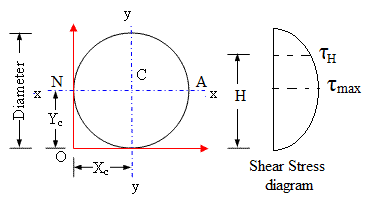# Describe and calculate the Bending Stresses in Square Section

Bending stress is a type of stress that occurs in a material when it is subjected to a bending moment. The bending moment is created by an external load that is applied to the material, causing it to bend. In the case of a square section, the bending stress will be highest at the corners, where the material is experiencing the most compression and tension.

To calculate the bending stress in a square section, we need to consider the following factors:

1. The size of the section (side length)
2. The material properties of the section (modulus of elasticity and moment of inertia)
3. The bending moment applied to the section

The formula for calculating the bending stress in a square section is:

σ = My/I

where:

σ = bending stress

M = bending moment

y = distance from the neutral axis to the point of interest

I = moment of inertia of the section

Steps to calculate the bending stress in a square section:

1. Determine the side length (a) of the square section.
2. Calculate the moment of inertia (I) of the section using the formula: I = a4/12
3. Determine the bending moment (M) applied to the section.
4. Calculate the distance from the neutral axis to the point of interest (y).
5. Use the formula σ = My/I to calculate the bending stress at the point of interest.

In conclusion, calculating the bending stress in a square section requires a thorough understanding of the section’s dimensions, material properties, and applied loads. The formula for bending stress is straightforward and can be used to calculate the stress at any point along the section, as long as the moment of inertia and bending moment are known. In a square section, the highest bending stress will occur at the corners due to the maximum compression and tension experienced there.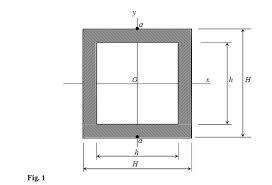# Describe and calculate Bending Stress in Triangular Section

Bending stress is a type of stress that occurs in a material when it is subjected to a bending moment. The bending moment is created by an external load that is applied to the material, causing it to bend. In the case of a triangular section, the bending stress will be highest at the corners, where the material is experiencing the most compression and tension.

To calculate the bending stress in a triangular section, we need to consider the following factors:

1. The size of the section (base and height)
2. The material properties of the section (modulus of elasticity and moment of inertia)
3. The bending moment applied to the section

The formula for calculating the bending stress in a triangular section is:

σ = My/I

where:

σ = bending stress

M = bending moment

y = distance from the neutral axis to the point of interest

I = moment of inertia of the section

Steps to calculate the bending stress in a triangular section:

1. Determine the base (b) and height (h) of the triangular section.
2. Calculate the moment of inertia (I) of the section using the formula: I = bh3/36
3. Determine the bending moment (M) applied to the section.
4. Calculate the distance from the neutral axis to the point of interest (y).
5. Use the formula σ = My/I to calculate the bending stress at the point of interest.

In conclusion, calculating the bending stress in a triangular section requires a thorough understanding of the section’s dimensions, material properties, and applied loads. The formula for bending stress is straightforward and can be used to calculate the stress at any point along the section, as long as the moment of inertia and bending moment are known. In a triangular section, the highest bending stress will occur at the corners due to the maximum compression and tension experienced there.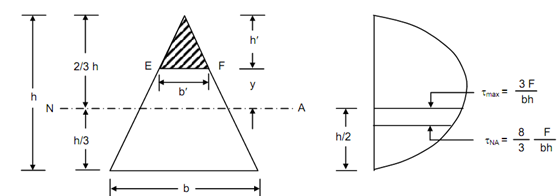# Describe and calculate Bending Stresses in I (symmetrical and unsymmetrical) section

Bending stress is a type of stress that occurs in a material when it is subjected to a bending moment. The bending moment is created by an external load that is applied to the material, causing it to bend. In the case of an I-section, the bending stress will be highest at the outer fibers of the web, where the material is experiencing the most compression and tension.

To calculate the bending stress in an I-section, we need to consider the following factors:

1. The size of the section (flange width, flange thickness, web height, and web thickness)
2. The material properties of the section (modulus of elasticity and moment of inertia)
3. The bending moment applied to the section

The formula for calculating the bending stress in an I-section is:

σ = My/I

where:

σ = bending stress

M = bending moment

y = distance from the neutral axis to the point of interest

I = moment of inertia of the section

Steps to calculate the bending stress in an I-section:

1. Determine the dimensions of the section (flange width, flange thickness, web height, and web thickness).
2. Calculate the moment of inertia (I) of the section using the appropriate formula for symmetrical or unsymmetrical sections.
3. Determine the bending moment (M) applied to the section.
4. Calculate the distance from the neutral axis to the point of interest (y).
5. Use the formula σ = My/I to calculate the bending stress at the point of interest.

In conclusion, calculating the bending stress in an I-section requires a thorough understanding of the section’s dimensions, material properties, and applied loads. The formula for bending stress is straightforward and can be used to calculate the stress at any point along the section, as long as the moment of inertia and bending moment are known. In an I-section, the highest bending stress will occur at the outer fibers of the web due to the maximum compression and tension experienced there.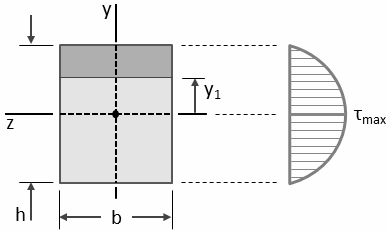# Describe and calculate Bending Stresses in T-section

Bending stress is a type of stress that occurs in a material when it is subjected to a bending moment. The bending moment is created by an external load that is applied to the material, causing it to bend. In the case of a T-section, the bending stress will be highest at the outer fibres of the flange, where the material is experiencing the most compression and tension.

To calculate the bending stress in a T-section, we need to consider the following factors:

1. The size of the section (flange width, flange thickness, web height, and web thickness)
2. The material properties of the section (modulus of elasticity and moment of inertia)
3. The bending moment applied to the section

The formula for calculating the bending stress in a T-section is:

σ = My/I

where:

σ = bending stress

M = bending moment

y = distance from the neutral axis to the point of interest

I = moment of inertia of the section

Steps to calculate the bending stress in a T-section:

1. Determine the dimensions of the section (flange width, flange thickness, web height, and web thickness).
2. Calculate the moment of inertia (I) of the section using the appropriate formula for T-sections.
3. Determine the bending moment (M) applied to the section.
4. Calculate the distance from the neutral axis to the point of interest (y).
5. Use the formula σ = My/I to calculate the bending stress at the point of interest.

In conclusion, calculating the bending stress in a T-section requires a thorough understanding of the section’s dimensions, material properties, and applied loads. The formula for bending stress is straightforward and can be used to calculate the stress at any point along the section, as long as the moment of inertia and bending moment are known. In a T-section, the highest bending stress will occur at the outer fibers of the flange due to the maximum compression and tension experienced there.

# Explain Comparison between Rectangular Beam

1. Strength: Rectangular beams are strong in the direction of their width. The width of a rectangular beam provides a large area for the load to be distributed over, reducing the stress on any one point and increasing the overall strength of the beam.
2. Stiffness: Rectangular beams are also stiff in the direction of their width. Stiffness refers to the ability of a beam to resist deformation under a load. The larger the width of a rectangular beam, the stiffer it will be, as the width provides more material to resist deformation.
3. Stress distribution: The stress distribution in a rectangular beam is uniform along the length of the beam. This means that the stress experienced by the beam is evenly distributed over its entire length, reducing the risk of failure at any one point.

It is important to note that while rectangular beams are strong, stiff, and have a uniform stress distribution, they may not be the best choice for every situation. The choice of beam shape will depend on the specific requirements of a particular project, such as the size and type of load being applied, the available space, and the overall cost.

In conclusion, rectangular beams are a common choice for construction due to their strength, stiffness, and uniform stress distribution. When comparing rectangular beams to other beam shapes, it is important to consider the specific requirements of the project and choose the shape that will best meet those needs.

# Explain Comparison between Square Beams

1. Strength: Square beams have a higher strength-to-weight ratio than rectangular beams with the same cross-sectional area. This means that square beams are stronger for their size compared to rectangular beams, making them a good choice when weight is a concern.
2. Stiffness: Square beams are also stiffer than rectangular beams with the same cross-sectional area. The equal height and width of square beams means that they resist deformation more effectively than rectangular beams, making them a good choice when stiffness is a concern.
3. Stress distribution: The stress distribution in a square beam is uniform along the length and width of the beam. This means that the stress experienced by the beam is evenly distributed over its entire area, reducing the risk of failure at any one point.

It is important to note that while square beams have some advantages over rectangular beams, they may not be the best choice for every situation. The choice of beam shape will depend on the specific requirements of a particular project, such as the size and type of load being applied, the available space, and the overall cost.

In conclusion, square beams are a type of rectangular beam that offer higher strength and stiffness compared to rectangular beams with the same cross-sectional area. However, they may not be the best choice for every situation and the choice of beam shape will depend on the specific requirements of a project.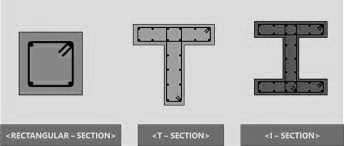# Explain Comparison between Rectangular and circular Beams

1. Strength: Circular beams have a higher strength-to-weight ratio than rectangular beams with the same cross-sectional area. This means that circular beams are stronger for their size compared to rectangular beams, making them a good choice when weight is a concern.
2. Stiffness: Circular beams are also stiffer than rectangular beams with the same cross-sectional area. The circular shape of a beam provides a uniform distribution of material around the entire circumference, which makes it more resistant to deformation under load compared to a rectangular beam.
3. Stress distribution: The stress distribution in a circular beam is more uniform compared to a rectangular beam. The circular shape of the beam means that stress is evenly distributed around the entire circumference, reducing the risk of failure at any one point.

It is important to note that while circular beams have some advantages over rectangular beams, they may not be the best choice for every situation. The choice of beam shape will depend on the specific requirements of a particular project, such as the size and type of load being applied, the available space, and the overall cost.

In conclusion, circular beams offer advantages in terms of strength and stiffness compared to rectangular beams with the same cross-sectional area. However, they may not be the best choice for every situation and the choice of beam shape will depend on the specific requirements of a project.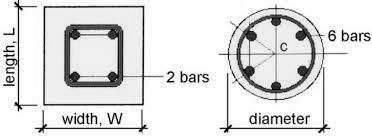# Explain the concept of Beam of Uniform Strength

The concept of a beam of uniform strength refers to a beam that has a constant cross-sectional area throughout its length. This means that the strength and stiffness of the beam are consistent along its entire length, and it experiences uniform stress distribution under load.

In a beam of uniform strength, the cross-sectional area does not change, and the distribution of material is consistent along the length of the beam. This means that the beam can withstand a uniform load without experiencing any variations in stress or deflection.

The concept of a beam of uniform strength is important in engineering and design because it provides a simple and straightforward way of determining the strength and stiffness of a beam. It is also a useful starting point for designing more complex beams with varying cross-sectional areas, as it provides a baseline for comparison.

It is worth noting that in real-world applications, it is not always possible to have a beam of uniform strength. For example, a beam may need to be designed with varying cross-sectional areas to accommodate changes in load or to accommodate other design requirements. In these cases, the design must take into account the changes in strength and stiffness along the length of the beam to ensure its overall stability and reliability.

In conclusion, the concept of a beam of uniform strength refers to a beam that has a constant cross-sectional area and uniform material distribution throughout its length. This provides a simple and straightforward way of determining the strength and stiffness of a beam and is a useful starting point for designing more complex beams.

# Explain the phenomena of Eccentric Loading on the Cross-section of the Beam

The cross-section of the beam is affected by eccentric loading in several ways. Firstly, the bending moment acting on the beam is increased, leading to an increase in the magnitude of bending stress. Secondly, the point of maximum stress along the cross-section of the beam shifts from the center of the cross-section to the side closest to the eccentric load.

In addition, eccentric loading also causes torsional stress, which results from the twisting of the beam about its longitudinal axis. Torsional stress can lead to failure in the beam, especially in beams with a low torsional stiffness, such as circular or oval beams.

The magnitude of bending and torsional stress caused by eccentric loading can be calculated using classical mechanics and strength of materials theories. The calculation takes into account the size and shape of the cross-section of the beam, the magnitude of the eccentric load, and the position of the load relative to the center of the beam.

In conclusion, eccentric loading refers to a type of loading where the load is offset from the center of a beam. Eccentric loading causes both bending and torsional stress and affects the cross-section of the beam by increasing the magnitude of bending stress and shifting the point of maximum stress. The effects of eccentric loading can be calculated using classical mechanics and strength of materials theories.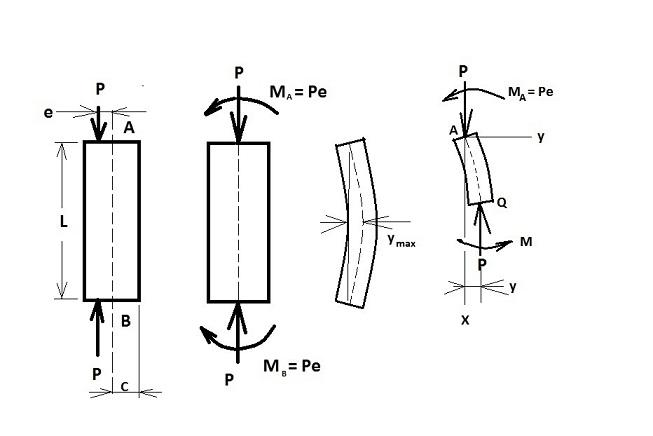# Explain the Middle One-Third Rule for Rectangular Section

The middle one-third rule is a rule used to determine the maximum stress in a rectangular cross-section beam under bending. The rule states that, under pure bending, the maximum bending stress occurs at a point one-third of the distance from the neutral axis to the outer surface of the beam, and the maximum stress is proportional to the distance from this point to the neutral axis.

The neutral axis of a beam is defined as the axis along which the cross-section experiences no net change in length as the beam is bent. In other words, the neutral axis is the axis about which the cross-section of the beam remains unchanged during bending.

The middle one-third rule is based on the observation that, for most practical purposes, the distribution of stress along the cross-section of a beam is proportional to the distance from the neutral axis. The rule assumes that the maximum stress occurs at a point one-third of the way from the neutral axis to the outer surface of the beam.

It is important to note that the middle one-third rule is an approximation and may not be accurate in all cases, especially for beams with complex cross-sectional shapes or for beams under more complex loading conditions. In these cases, more sophisticated analytical methods may need to be used to accurately predict the distribution of stress along the cross-section of the beam.

In conclusion, the middle one-third rule is a rule used to determine the maximum stress in a rectangular cross-section beam under bending. The rule states that the maximum stress occurs at a point one-third of the distance from the neutral axis to the outer surface of the beam and that the maximum stress is proportional to the distance from this point to the neutral axis. The rule is an approximation and may not be accurate in all cases, and more sophisticated methods may be needed for more complex cases.

# Explain the Middle One-Fourth Rule for Circular Section

The middle one-fourth rule is a rule used to determine the maximum stress in a circular cross-section beam under bending. The rule states that, under pure bending, the maximum bending stress occurs at a point one-fourth of the distance from the neutral axis to the outer surface of the beam, and the maximum stress is proportional to the distance from this point to the neutral axis.

The neutral axis of a beam is defined as the axis along which the cross-section experiences no net change in length as the beam is bent. In other words, the neutral axis is the axis about which the cross-section of the beam remains unchanged during bending.

The middle one-fourth rule is based on the observation that, for most practical purposes, the distribution of stress along the cross-section of a circular beam is proportional to the distance from the neutral axis. The rule assumes that the maximum stress occurs at a point one-fourth of the way from the neutral axis to the outer surface of the beam.

It is important to note that the middle one-fourth rule is an approximation and may not be accurate in all cases, especially for beams with complex cross-sectional shapes or for beams under more complex loading conditions. In these cases, more sophisticated analytical methods may need to be used to accurately predict the distribution of stress along the cross-section of the beam.

In conclusion, the middle one-fourth rule is a rule used to determine the maximum stress in a circular cross-section beam under bending. The rule states that the maximum stress occurs at a point one-fourth of the distance from the neutral axis to the outer surface of the beam and that the maximum stress is proportional to the distance from this point to the neutral axis. The rule is an approximation and may not be accurate in all cases, and more sophisticated methods may be needed for more complex cases.

# Explain the concept of Composite Beams/Flitched Beam

Composite beams, also known as flitched beams, are beams made up of multiple materials or components that are combined to form a single structural element. These beams are used in construction to combine the strengths of different materials to create a beam that is stronger, lighter, or has other desirable properties than a beam made of a single material.

Composite beams typically consist of two or more materials, with each material chosen for its specific properties. For example, a composite beam might have a concrete slab and a steel beam joined together to form a single beam. The concrete provides the compressive strength and stiffness, while the steel provides the tensile strength and resistance to bending.

The components of a composite beam are joined together in a variety of ways, including adhesion, bonding, or mechanical fastening. In some cases, the components are also connected by specialised connectors, such as shear connectors, which transfer shear forces between the two components.

One advantage of composite beams is that they allow for the combination of different materials to create a beam that is stronger and lighter than a beam made of a single material. Additionally, composite beams can also be designed to be more resistant to cracking or other forms of failure than beams made of a single material.

Another advantage of composite beams is that they can be used to retrofit existing structures. For example, an existing concrete beam might be strengthened by adding a steel flange to the top and bottom of the beam, which increases its resistance to bending and cracking.

In conclusion, composite beams, or flitched beams, are beams made up of multiple materials or components that are combined to form a single structural element. These beams offer a number of advantages, including increased strength and stiffness, lighter weight, and resistance to cracking or other forms of failure. Additionally, composite beams can also be used to retrofit existing structures.